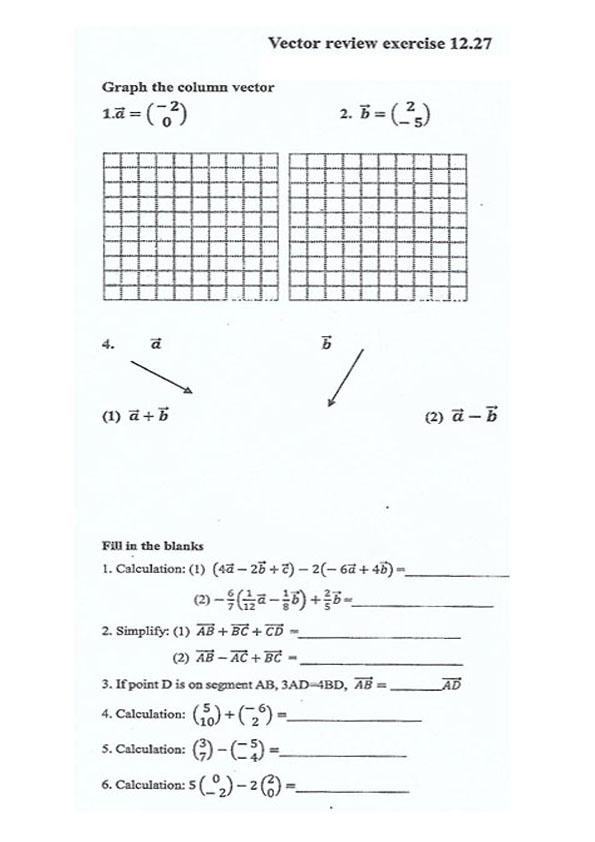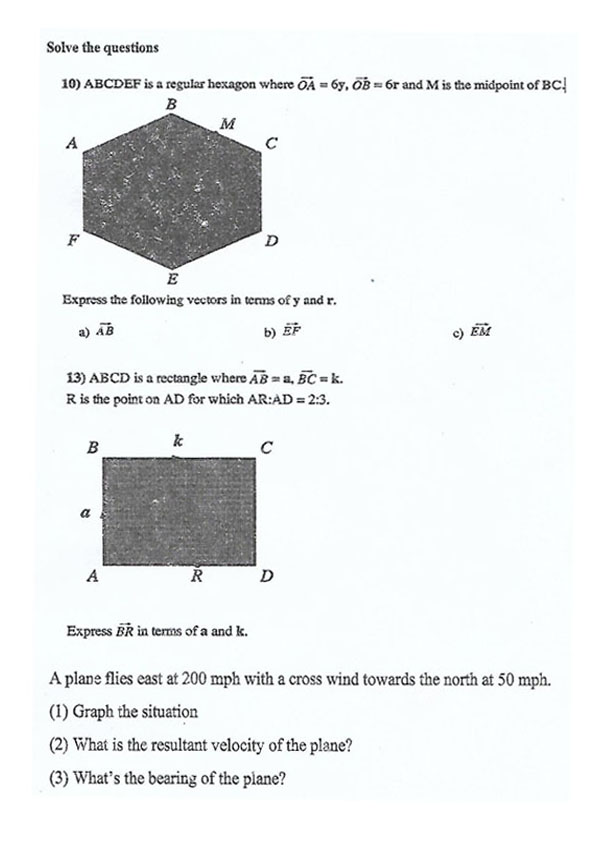## 上海尚德国际学校9年级数学练习题

#### Applications of Ouadratic Functions 12.20

1. A manufacturer of fax machines find that the cost(in dollars) generated by manufacturing x units per week is given by the function C(x)=0.15x²-39x+4500. How many units should be manufactured to minimize the cost?

2. The main cable of a suspension bridge has the shape of a parabola. The cables are strung from the tops of two towers, 200 feet apart, each 50 feet high. The cable is 5 feet above the roadway at the point that is directly between the towers. A. Determine a function for the parabola. B. How long is the vertical support cable that is 40 feet from one of the towers?

3. The arch of a doorway is in the shape of a parabola. The bottom of the arch is 4 feet above the floor, and the top of the arch is 8 feet above the floor.The doorway is 4 feet wide A. Determine the function for the parabola. B. Is it possible to pass a box with dimensions 6×3×10 feet through the doorway?

4. What is the greatest area of a pasture in the shape of a rectangle that can be fenced with 6000 feet of fencing?

5. Suppose that f(x)=x²-kx+16. Determine a value for k such that the graph of f

has: A.No x-intercepts. B.Exactly one x-intercept. C.Two x-intercepts.

6. Suppose that f(x)=x²-4x+c. Determine a value for c such that the graph of f  has:

A.No x-intercepts. B.Exactly one x-intercept. C.Two x-intercepts.

7. A cylindrical alurninum can is being constructed to have a height h of 4 inches (see figure). If the can is to have a volume of 28 cubic inches, approximate its radiusr
(Hint:V=πr²h.)